Milk can be classified in terms of its quality into three groups: low quality, medium quality, and high quality.

In this example, we will predict milk quality by seven observable milk variables.

The central goal is to design a model that makes proper classifications for new milk samples. In other words, one which exhibits good generalization.

### Contents

This example is solved with Neural Designer. To follow it step by step, you can use the free trial.

## 1. Application type

This is a classification project. Indeed, the variable to be predicted is categorical (low, medium and high).

The objective is to model the quality of the milk by knowing its characteristics and thus be able to make future predictions.

## 2. Data set

The first step is to prepare the data set. This is the source of information for the classification problem. For that, we need to configure the following concepts:

• Data source.
• Variables.
• Instances.

### Data source

The data source is the file milk.csv. It contains the data for this example in comma-separated values (CSV) format. The number of columns is 8, and the number of rows is 1060.

### Variables

The variables are:

• pH: This feature defines pH of the milk, which is in the range of 3 to 9.5.
• temperature: This feature defines the temperature of the milk, and its range is from 34’C to 90’C.
• taste: This feature defines the taste of the milk and takes the possible values: 1 (good) or 0 (bad).
• odor: This feature defines the odor of the milk and takes the possible values: 1 (good) or 0 (bad).
• fat: This feature defines the fat of the milk and takes the possible values: 1 (good) or 0 (bad).
• turbidity: This feature defines the turbidity of the milk and takes the possible values: 1 (good) or 0 (bad).
• colour: This feature defines the color of the milk, which is in the range of 240 to 255.
• grade: This is the target and takes the values: low_quality, medium_quality, or high_quality.

All variables in the study are inputs, except “grade”, which is the output that we want to extract from this machine learning study. Note that “grade” is categorical and can take the values low_quality, medium_quality, and high_quality.

### Instances

The instances are divided into training, selection, and testing subsets. They represent 60.2% (637), 19.9% (211), and 19.9% (211) of the original instances, respectively, and are randomly split.

The milk dataset contains 429 instances of low quality, 374 instances of medium quality, and 256 instances of high quality. The next figure is the pie chart for the variable milk quality class, and it shows its distribution.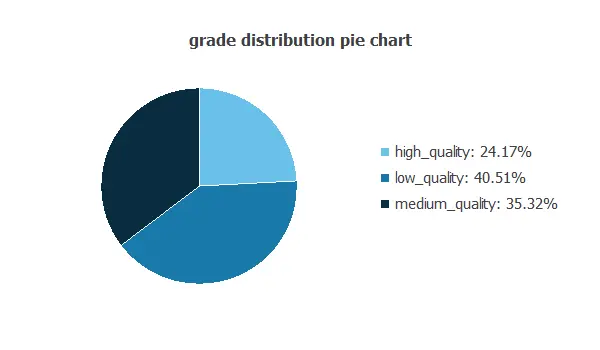As we can see, the target is not well-distributed. Indeed, there are more samples of low_quality, with 40.5 % of the total samples and only 24.17% of the total samples of high_quality.

## 3. Neural network

The second step is to choose a neural network. For classification problems, it is usually composed by:

• A scaling layer.
• A perceptron layers.
• A probabilistic layer.

The neural network must have seven inputs since the data set has seven input variables.

The scaling layer normalizes the input values. As our inputs have different distributions, they are scaled with different methods:

In this case, as first guest we only use one perceptron layer. This layer contains seven inputs, three neurons, and three outputs. For this example, the perceptron layer is a hyperbolic tangent activation function.

The probabilistic layer allows to interpret the outputs as probabilities. In this regard, all outputs are between 0 and 1, and their sum is 1. The softmax probabilistic activation is used here.

The neural network has three outputs because we have three different “grades”: low_quality, medium_quality and high_quality.

The next figure is a graphical representation of this classification neural network: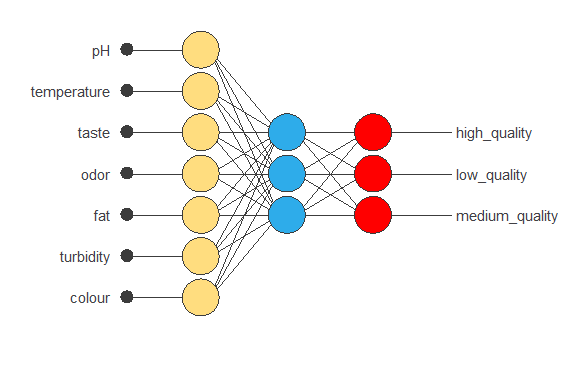## 4. Training strategy

The next step is to set the training strategy, which is composed of:

• Loss index.
• Optimization algorithm.

The loss index chosen for this application is the normalized squared error with L2 regularization.

The error term fits the neural network to the training instances of the data set. The regularization term makes the model more stable and improves generalization.

The optimization algorithm searches for the neural network parameters that minimize the loss index. The quasi-Newton method is chosen here.

The following chart shows how the training and selection errors decrease with the epochs during training.

The final values are training error = 0.252 NSE (blue), and selection error = 0.277 NSE (orange).Is it important to have low selection error in our model, allowing us to generalize well the new data rather than simply memorizing the training data.

## 5. Model selection

The objective of model selection is to find the network architecture with the best generalization properties. That is, that which minimizes the error on the selected instances of the data set.

We want to find a neural network with a selection error of less than 0.277 NSE, which is the value we have achieved so far.

Order selection algorithms train several network architectures with a different number of neurons and select that with the smallest selection error.

The incremental order method starts with a small number of neurons and increases the complexity at each iteration. The following chart shows the training error (blue) and the selection error (yellow) as a function of the number of neurons.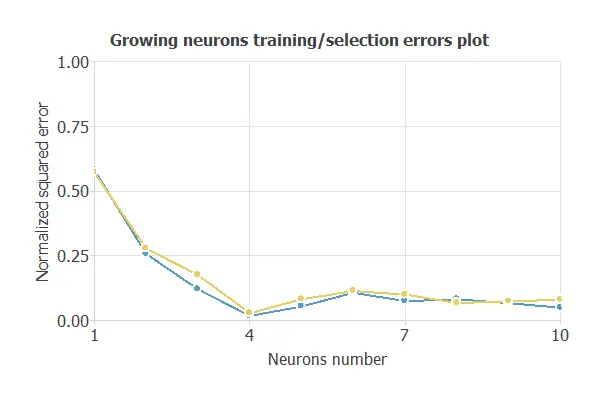As we can see, the number of neurons that yield the minimum error is four. Therefore, we select the neural network with four neurons in the perceptron layer. The next chart show the new neural network architecture.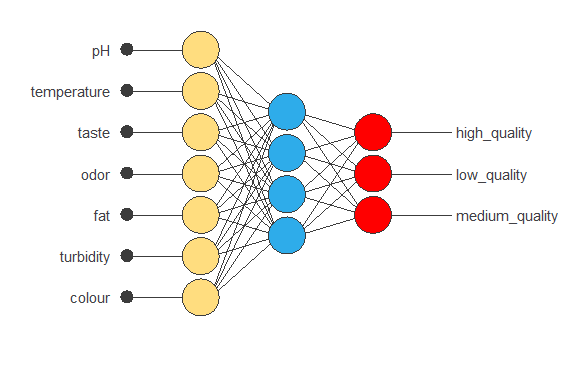The following chart shows how the training and selection errors decrease with the epochs during training in the new neural network. The final values are training error = 0.0877 NSE (blue), and selection error = 0.107 NSE (orange).

With the new architecture of the neural network, we achieve around 50% less selection error.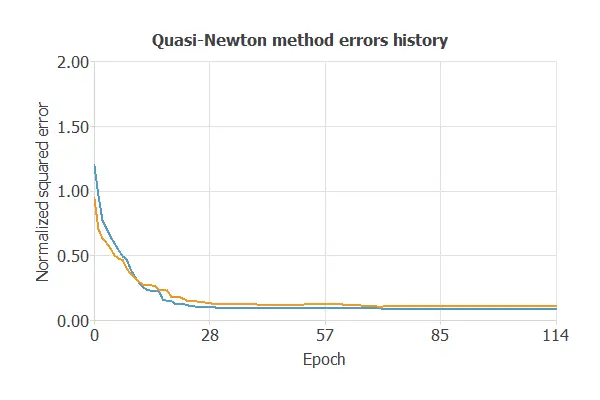## 6. Testing analysis

The purpose of the testing analysis is to validate the generalization performance of the model. Here we compare the neural network outputs to the corresponding targets in the testing instances of the data set.

In the confusion matrix, the rows represent the targets (or real values) and the columns the corresponding outputs (or predictive values).

Predicted low_quality Predicted medium_quality Predicted high_quality Total
Real low_quality 49 (23.2%) 0 4 (1.9%) 53 (23.7%)
Real medium_quality 1 (0.5%) 92 (43.6%) 0 93 (43.6%)
Real high_quality 0 0 65 (30.8%) 65 (32.7%)
Total 50 (23.7%) 92 (43.6%) 69 (32.7%) 211 (100%)

The number of correctly classified samples is 206, and the number of misclassified samples is 5.

The confusion matrix allows us to calculate the model’s accuracy and error:

• Classification accuracy: 97.6%.
• Error rate: 2.4%.

## 7. Model deployment

The neural network is now ready to predict outputs for inputs that it has never seen. This process is called model deployment.

To classify a sample of milk, we calculate the neural network outputs. For instance, consider a sample with the following features:

• ph: 6.63
• temperature: 39.2
• taste: 0
• odor: 1
• fat: 1
• turbidity: 1

The neural network outputs for this features are:

• high_quality: 91.2%
• medium_quality: 8.73%
• low_quality: 0.07%

For this particular case, the neural network would classify the sample of milk as being of high_quality since it has the highest probability.

The mathematical expression of the trained neural network is listed below.

scaled_pH = (pH-6.630119801)/1.399680018;
scaled_temperature = (temperature-44.22660065)/10.09840012;
scaled_taste = taste*(1+1)/(1-(0))-0*(1+1)/(1-0)-1;
scaled_odor = odor*(1+1)/(1-(0))-0*(1+1)/(1-0)-1;
scaled_fat = fat*(1+1)/(1-(0))-0*(1+1)/(1-0)-1;
scaled_turbidity = turbidity*(1+1)/(1-(0))-0*(1+1)/(1-0)-1;
scaled_colour = (colour-251.8399963)/4.30742979;

perceptron_layer_1_output_0 = tanh( -0.058706 + (scaled_pH*-3.09864) + (scaled_temperature*-2.08141) + (scaled_taste*0.0928608) + (scaled_odor*1.25385) + (scaled_fat*2.07282) + (scaled_turbidity*0.00868495) + (scaled_colour*0.344164) );
perceptron_layer_1_output_1 = tanh( 0.23707 + (scaled_pH*-0.124321) + (scaled_temperature*2.62452) + (scaled_taste*0.903015) + (scaled_odor*0.730532) + (scaled_fat*0.447419) + (scaled_turbidity*0.235073) + (scaled_colour*1.864) );
perceptron_layer_1_output_2 = tanh( 1.37137 + (scaled_pH*3.56574) + (scaled_temperature*-5.7119) + (scaled_taste*-0.719459) + (scaled_odor*0.622088) + (scaled_fat*-1.22018) + (scaled_turbidity*-1.07232) + (scaled_colour*-0.576336) );
perceptron_layer_1_output_3 = tanh( -0.937949 + (scaled_pH*5.19285) + (scaled_temperature*-1.96228) + (scaled_taste*0.552014) + (scaled_odor*1.22828) + (scaled_fat*1.60562) + (scaled_turbidity*1.39285) + (scaled_colour*0.712061) );

probabilistic_layer_combinations_0 = -2.21108 +3.43582*perceptron_layer_1_output_0 -0.0137288*perceptron_layer_1_output_1 -0.730089*perceptron_layer_1_output_2 +5.37976*perceptron_layer_1_output_3
probabilistic_layer_combinations_1 = 2.80993 -2.64362*perceptron_layer_1_output_0 +1.6769*perceptron_layer_1_output_1 -2.2073*perceptron_layer_1_output_2 -0.471236*perceptron_layer_1_output_3
probabilistic_layer_combinations_2 = -0.469866 -0.676177*perceptron_layer_1_output_0 -1.55635*perceptron_layer_1_output_1 +2.90799*perceptron_layer_1_output_2 -4.70235*perceptron_layer_1_output_3

sum = exp(probabilistic_layer_combinations_0) + exp(probabilistic_layer_combinations_1) + exp(probabilistic_layer_combinations_2);

high_quality = exp(probabilistic_layer_combinations_0)/sum;
low_quality = exp(probabilistic_layer_combinations_1)/sum;
medium_quality = exp(probabilistic_layer_combinations_2)/sum;


We can implement this expression in any programming language to obtain the output for our input.

## References

• Kaggle. Machine learning and data science community: Milk Dataset.